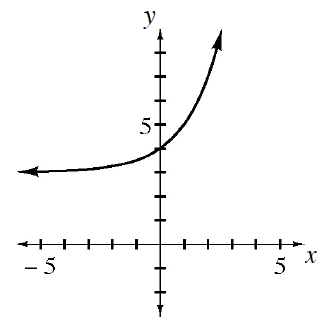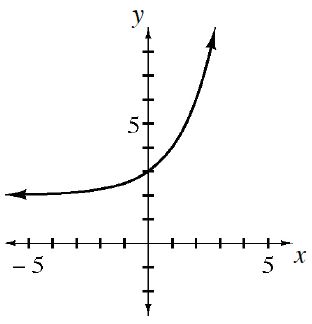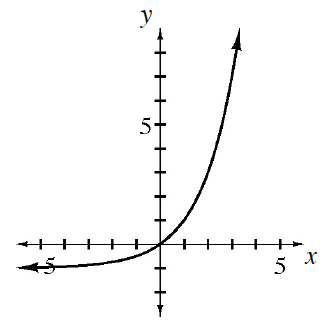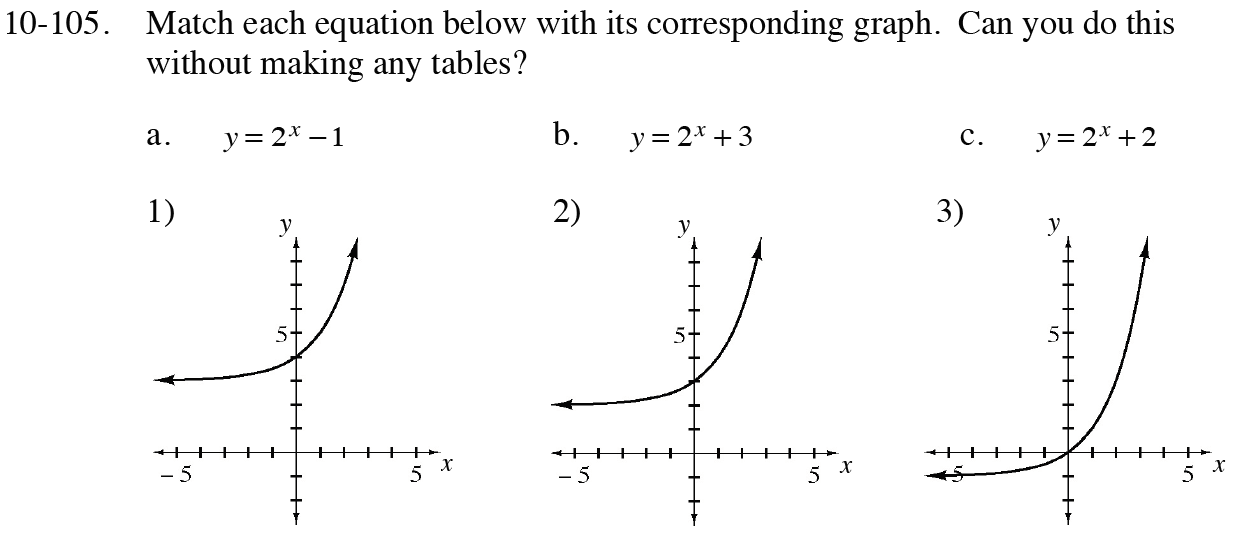### Home > CC4 > Chapter 10 > Lesson 10.2.3 > Problem10-105

10-105.
1. ​ Match each equation below with its corresponding graph. Can you do this without making any tables?​ Homework Help ✎

2.  a. y = 2x – 1 b. y = 2x + 3 c. y = 2x + 2 1)2)3)Consider the y-intercept for each function.

Considering equation (a). The y-intercept is calculated by letting x = 0.
So y = 20−1 so y = 0 when x = 0.
Which graph has (0,0) on it?